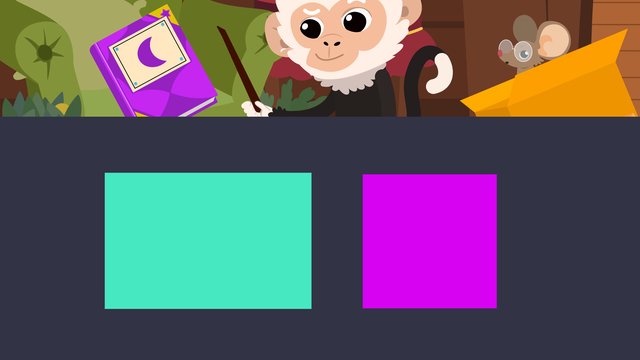# Quadrilaterals – Rectangles and Squares

Content Quadrilaterals – Rectangles and SquaresRating

Ø 4.7 / 7 ratings
The authorsTeam Digital
CCSS.MATH.CONTENT.3.G.A.1

## How to Identify a Rectangle and a Square

Do you know what quadrilaterals are? Quadrilaterals are everywhere! Can you see any around you? A window or the front of your TV are great examples of quadrilaterals, as they are squares and rectangles.

In this video and in the following text, you will learn the definition and properties of a rectangle in geometry. In addition, you will learn the definition and properties of a square.

A quadrilateral is a polygon with four straight sides. Any four sided shape you can think of is a quadrilateral. Is a rectangle shape a polygon that is a quadrilateral? Yes, it is! What about a square? It has four sides, so it is a quadrilateral too. Rectangles and squares are both a type of quadrilateral.

Shapes can be identified by their attributes or properties, as you will see below.

## What Is a Rectangle?

Per definition, a rectangle is a polygon that has four straight sides.

It is a quadrilateral because it has four sides. How many vertices does a rectangle have? The sides of a rectangle meet at a 90 degree angle, known as a right angle, and there are four of them. How many pairs of parallel sides does a rectangle have? A rectangle has two pairs of parallel sides that are equal in length.

### Properties of a Rectangle

So what does a rectangle actually look like? On the infographic below you can see a rectangle outline.Rectangles have two pairs of opposite sides which are equal in length. They also have four right angles.

## What Is a Square?

According to its definition, a square is a polygon that has four straight sides that are all equal in length.

It is a quadrilateral because it has four sides. How many vertices does a rectangle have? Just like the rectangle, the sides of a square meet at a 90 degree angle – as you now know the right angle – and there are also four of them. And how many pairs of parallel sides does a square have? A square also has two pairs of parallel sides that are all equal in length.

### Properties of a Square

So what does a square look like? On the infographic below you can see a square outline.Just like the rectangle, a square has four right angles. It also has two pairs of opposite sides, but they are all equal in length.

## Summary of Rectangles and SquaresRectangles and squares are both shapes that are quadrilaterals. Both of them have four straight sides and four right angles. While they are similar in properties, the main difference between a rectangle and a square is that a square has sides that are all the same length.

Properties of rectangles Properties of squares
four straight sides four straight sides
four right angles four right angles
two pairs of opposite sides equal in length all four sides equal in length

Would you like to practice some more? You can find exercises and worksheets about rectangles and squares on this page. Just click on the button “start exercises”. Enjoy!

## Quadrilaterals – Rectangles and Squares exercise

Would you like to apply the knowledge you’ve learned? You can review and practice it with the tasks for the video Quadrilaterals – Rectangles and Squares.
• ### Find the quadrilaterals in Nico's attic.

Hints

There are 5 quadrilaterals to find.

Solution
• There are 5 quadrilaterals in total: 2 squares and 3 rectangles.
• The rug, broom, blanket, and glasses are not quadrilaterals because they aren't polygons with 4 straight sides.
• ### Describe the different quadrilateral shapes.

Hints

Here are some examples of quadrilaterals.

Solution

A quadrilateral is a polygon with 4 straight sides. We learned that squares and rectangles are different types of quadrilaterals.

Squares are quadrilaterals with 4 equal sides and 4 right angles. Rectangles are quadrilaterals with 2 pairs of opposite sides that are equal, and 4 right angles. Every square is a special type of rectangle because it has 2 pairs of opposite sides that are equal in length.

• ### Identify the squares.

Hints

Make sure your shape is a quadrilateral that has 4 right angles.

Make sure your shape has 4 equal sides.

There are 3 squares.

Solution

The window, purple shape, and orange shape are all squares because they are all quadrilaterals that have 4 right angles and 4 equal sides.

The rest are all not squares.
The first shape is a rectangle because it has 2 pairs of equal sides.
The second shape is not a square because it isn't enclosed, therefore it isn't a polygon.
The third shape is not a square because it doesn't have 4 right angles.
The last shape is a rectangle because it has 2 pairs of equal sides.

• ### How many rectangles do you see?

Hints

Remember, rectangles are quadrilaterals that have 2 pairs of equal sides and 4 right angles.

Look at the shapes that might be rotated.

Remember, squares are a special type of rectangle.

Solution

There are 4 rectangles. 2 are special rectangles that are squares.
All 4 shapes have 2 pairs of equal sides and 4 right angles.

Hints

Solution

The square and rectangle are quadrilaterals because they both are polygons with 4 straight sides.

• ### Sort the shapes.

Hints

Remember, rectangles are quadrilaterals with 2 pairs of equal sides and 4 right angles.

Squares are both a square and also a special rectangle.

Solution

The squares are special rectangles, so they are both a rectangle and a square.
The rectangles have 2 pairs of equal sides and 4 right angles.
The triangle and parallelogram are neither a square or a rectangle.